# Energy & Power In Circuits

A. Electrical Power

Consider the circuit shown below: The battery gives the charges potential energy. In the bulb, electrical energy is transferred to thermal energy and then radiated. The battery supplies 5 J of energy every second, so its power is 5 watts. The bulb is taking energy at the same rate, so its power is also 5 watts.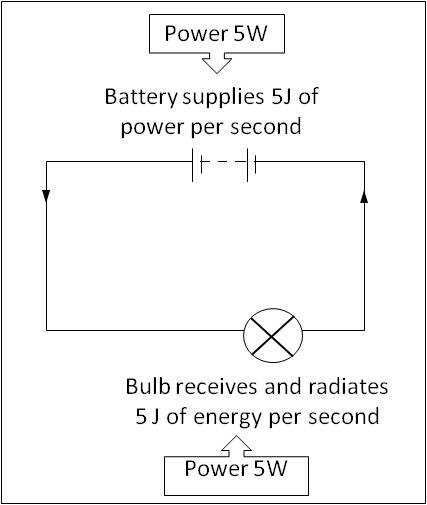Figure 1: Electrical Power

Power is the rate at which energy is transformed from one form to another. The SI unit of power is watt (W).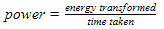Appliances such as refrigerators, televisions and ovens have a power rating marked on them, either in watts or in kilowatts.

B. Electrical Power Equation

In circuits, the power required for a component can be calculated by the potential difference across it and the current through it. The power is given by the following equation:C. Power Dissipated in a Resistor

When a current flows through a resistor, it has a heating effect. Electrons lose potential energy, which is changed into thermal energy. Hence, energy is dissipated in the resistor.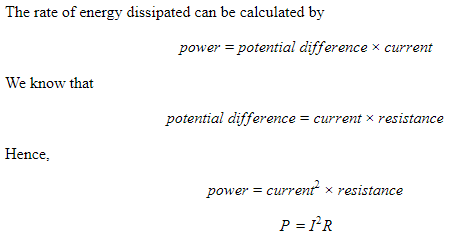D. Calculating Energy

Energy and power are linked by an equation. If the power of an appliance is known, the energy transformed at any given time can be calculated by the following equation.For example, if a 500 W heating element is switched on for 10 seconds. The energy transformed = 500 W × 10 s = 5000 J. So the heating element radiated 5000 J of thermal energy.

E. Electrical Energy Calculation

We know that P=VI, hence the equation of energy can be written as: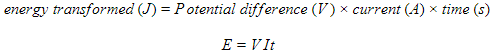F. Conservation of Energy (Kirchhoff’s Second Law)

A charge flowing through a circuit gains energy when it passes through a battery and loses energy when it passes through the rest of the circuit. According to the law of conservation of energy, the total energy must remain the same. The consequence of this conservation of energy is called Kirchhoff’s second law. It states that the sum of electromotive forces in a closed circuit is equal to the sum of the potential differences.

Let us consider the circuit shown in Figure 2. A battery is connected to a resistor and bulb in series.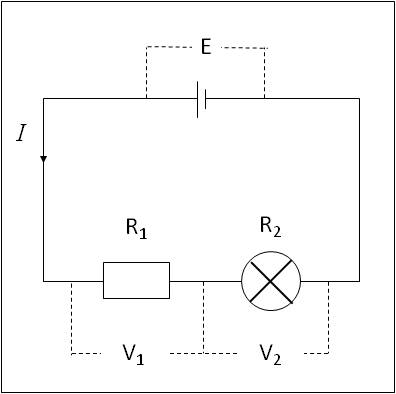Figure 2: An Electric Circuit

The electromotive force of a battery is E, the potential difference across resistor R1 is V1 and the potential difference across R2 is V2. Applying the law of conservation of energy, the electromotive force of battery is equal to the sum of the potential difference between the two resistances.G. Summary

• Electrical power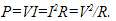• Energy = Power × time = VIt
• The unit for power is watt and the unit for energy is joules.
• In a closed loop of a circuit, the sum of electromotive forces is equal to the sum of the potential differences. This is Kirchhoff’s second law, and is a consequence of the law of conservation of energy.